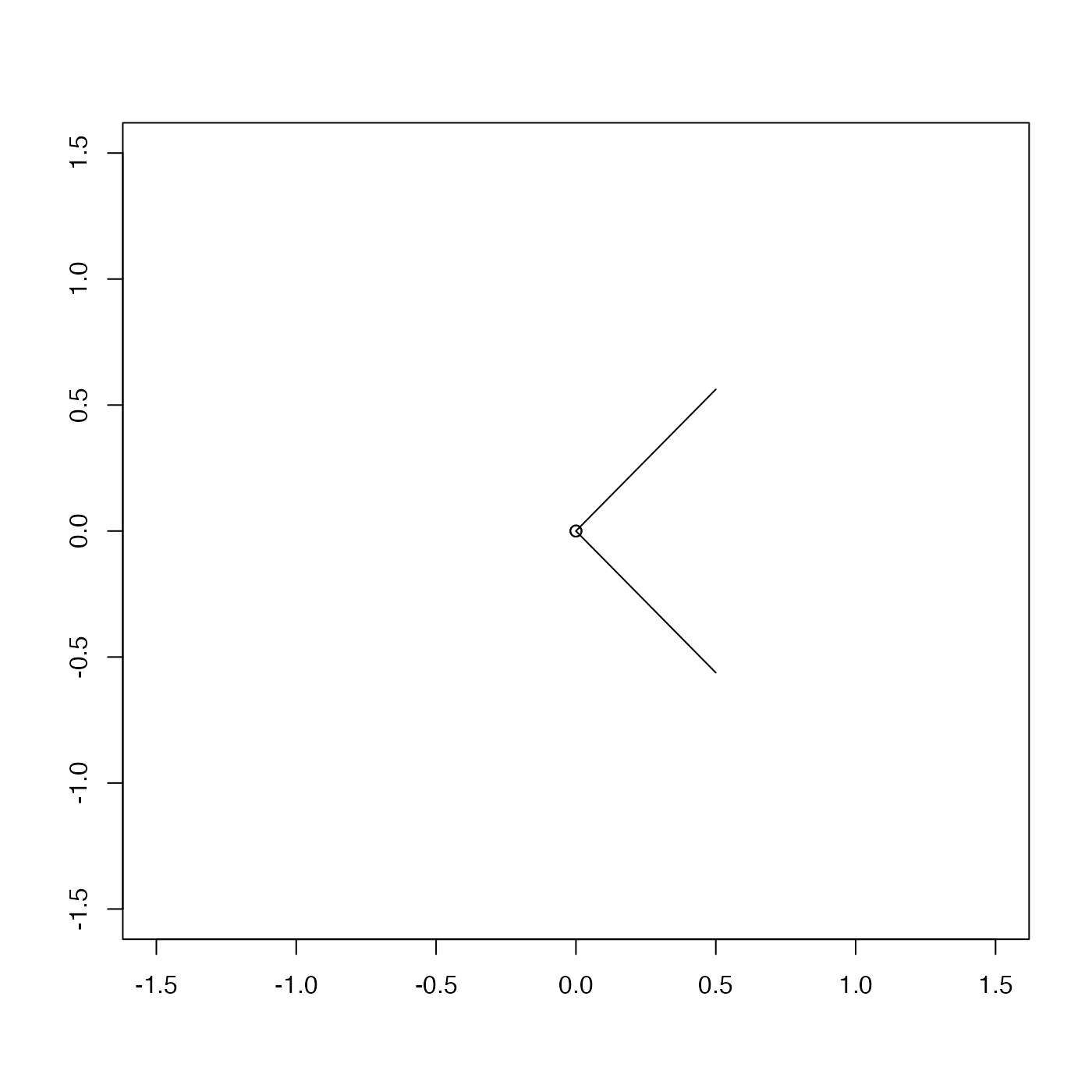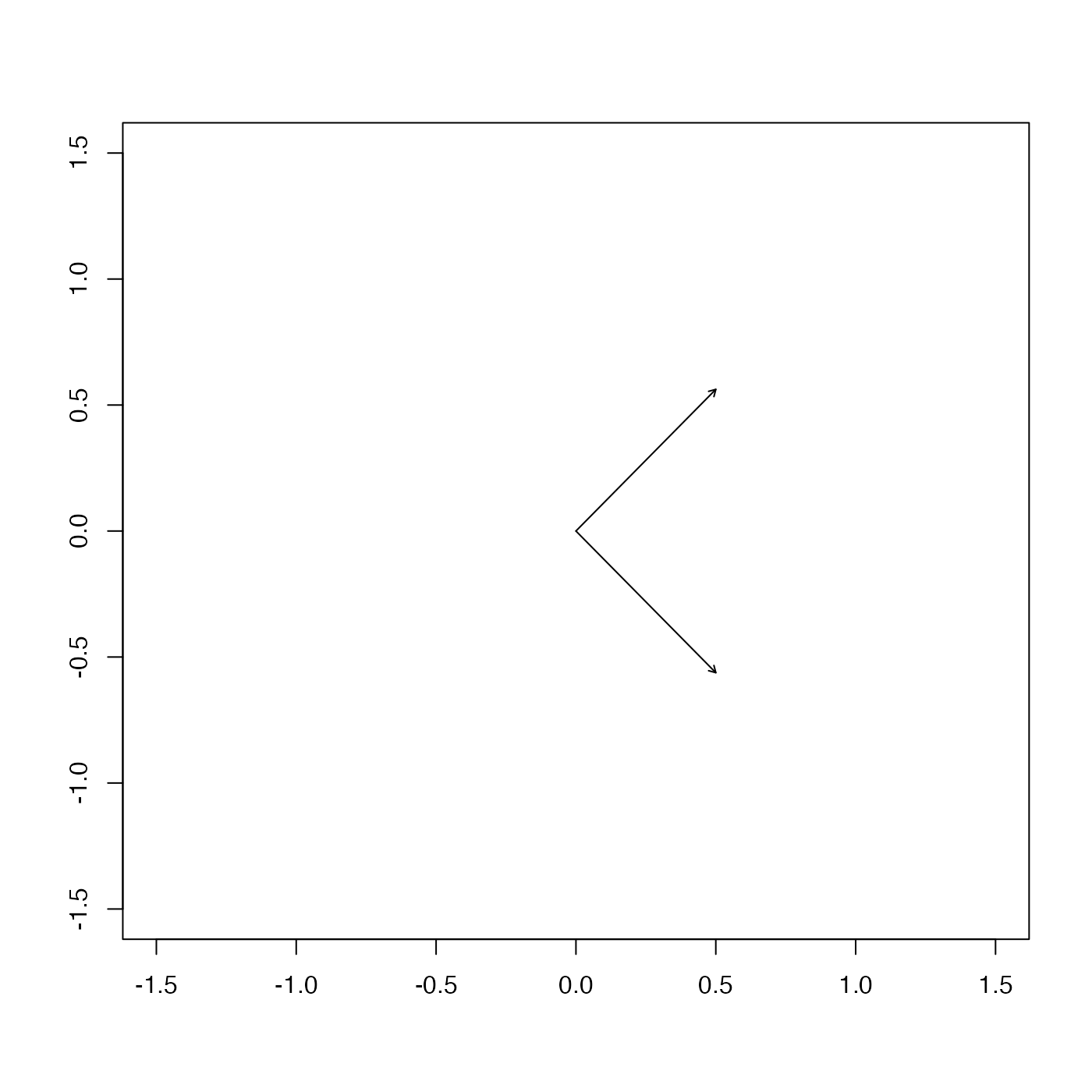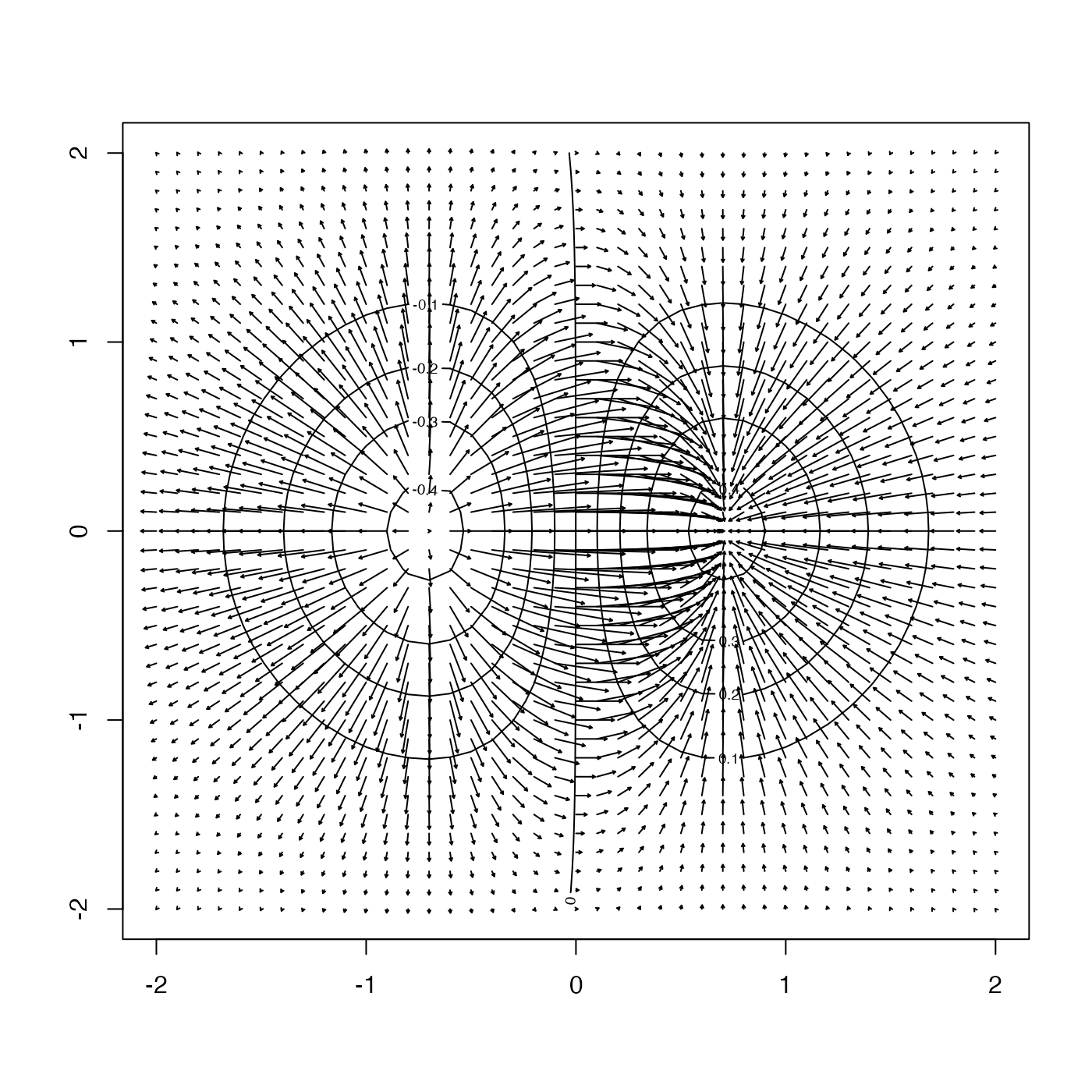The direction field is indicated variously, depending on the value of type:

drawDirectionField(
x,
y,
u,
v,
scalex,
scaley,
skip,
length = 0.05,
type = 1,
col = par("fg"),
pch = 1,
cex = par("cex"),
lwd = par("lwd"),
lty = par("lty"),
xlab = "",
ylab = "",
debug = getOption("oceDebug"),
...
)

## Arguments

x, y coordinates at which velocities are specified. The length of x and y depends on the form of u and v (vectors or matrices). velocity components in the x and y directions. Can be either vectors with the same length as x, y, or matrices, of dimension length(x) by length(y). scale to be used for the velocity arrows. Exactly one of these must be specified. Arrows that have u^2+v^2=1 will have length scalex along the x axis, or scaley along the y axis, according to which argument is given. either an integer, or a two-element vector indicating the number of points to skip when plotting arrows (for the matrix u, v case). If a single value, the same skip is applied to both the x and y directions. If a two-element vector, specifies different values for the x and y directions. indication of width of arrowheads. The somewhat confusing name of this argument is a consequence of the fact that it is passed to arrows() for drawing arrows. Note that the present default is smaller than the default used by arrows(). if TRUE, the arrows are added to an existing plot; otherwise, a new plot is started by calling plot() with x, y and type="n". In other words, the plot will be very basic. In most cases, the user will probably want to draw a diagram first, and add the direction field later. indication of the style of arrow-like indication of the direction. color of line segments or arrows; see par() for meaning plot character and expansion factor, used for type=1; see par() for meanings line width and type, used for type=2; see par() for meaning x and y axis labels debugging value; set to a positive integer to get debugging information. other arguments to be passed to plotting functions (e.g. axis labels, etc).

None.

## Details

• For type=1, each indicator is drawn with a symbol, according to the value of pch (either supplied globally, or as an element of the ... list) and of size cex, and color col. Then, a line segment is drawn for each, and for this lwd and col may be set globally or in the ... list.

• For type=2, the points are not drawn, but arrows are drawn instead of the line segments. Again, lwd and col control the type of the line.

## Examples

library(oce)
plot(c(-1.5, 1.5), c(-1.5, 1.5), xlab="", ylab="", type='n')drawDirectionField(x=rep(0, 2), y=rep(0, 2), u=c(1, 1), v=c(1, -1), scalex=0.5, add=TRUE)plot(c(-1.5, 1.5), c(-1.5, 1.5), xlab="", ylab="", type='n')drawDirectionField(x=rep(0, 2), y=rep(0, 2), u=c(1, 1), v=c(1, -1), scalex=0.5, add=TRUE,
type=2)## 2D example
x <- seq(-2, 2, 0.1)
y <- x
xx <- expand.grid(x, y)[,1]
yy <- expand.grid(x, y)[,2]
z <- matrix(xx*exp(-xx^2 -yy^2), nrow=length(x))
drawDirectionField(x, y, gz$gx, gz$gy, scalex=0.5, type=2, len=0.02)oceContour(x, y, z, add=TRUE)